# CSE 190 Neural Networks Gary Cottrell The Perceptron

• Slides: 20
Download presentation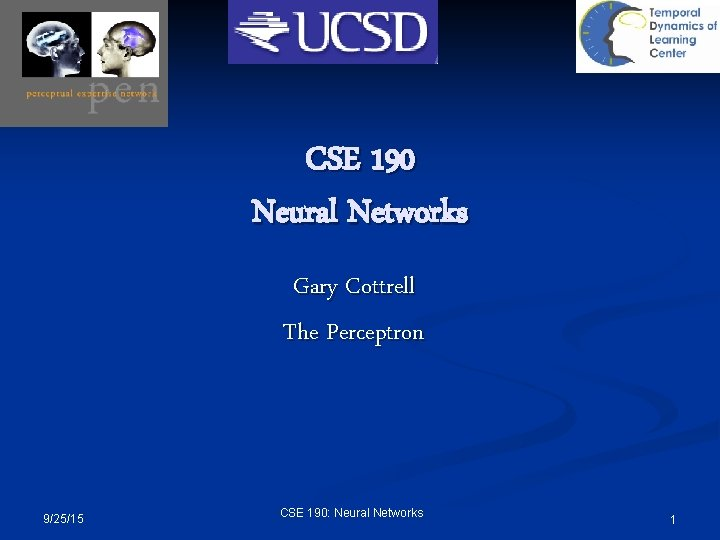CSE 190 Neural Networks Gary Cottrell The Perceptron 9/25/15 CSE 190: Neural Networks 1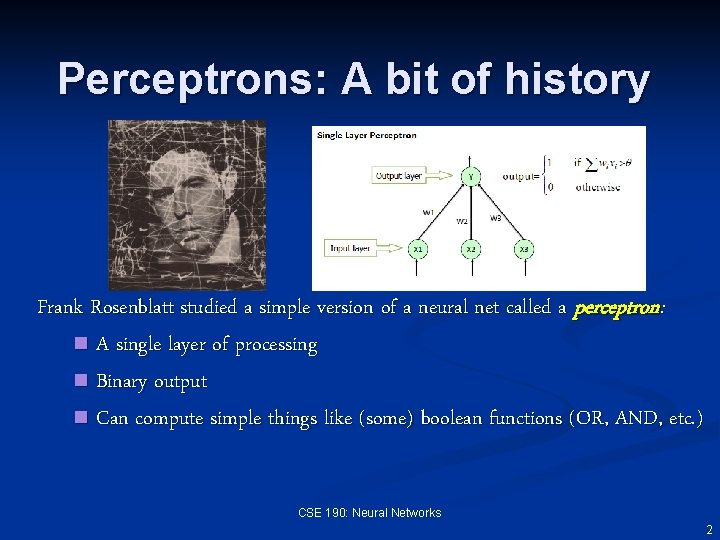Perceptrons: A bit of history Frank Rosenblatt studied a simple version of a neural net called a perceptron: n A single layer of processing n Binary output n Can compute simple things like (some) boolean functions (OR, AND, etc. ) CSE 190: Neural Networks 2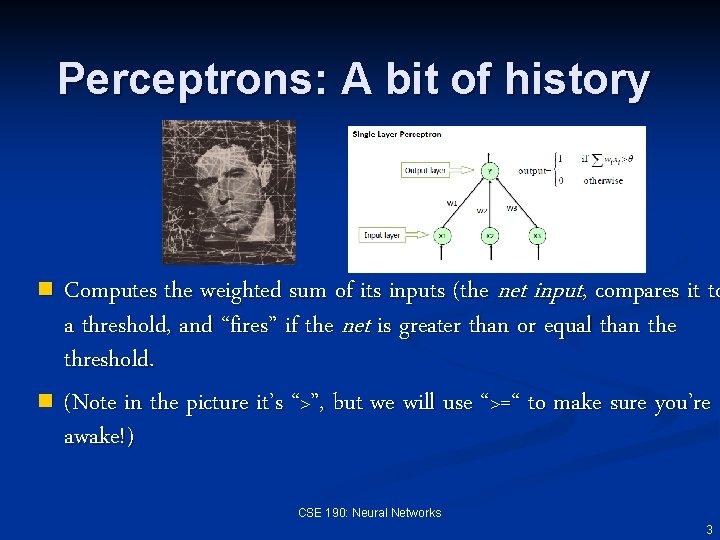Perceptrons: A bit of history Computes the weighted sum of its inputs (the net input, compares it to a threshold, and “fires” if the net is greater than or equal than the threshold. n (Note in the picture it’s “>”, but we will use “>=“ to make sure you’re awake!) n CSE 190: Neural Networks 3The Perceptron Activation Rule output net input This is called a linear threshold unit CSE 190: Neural Networks 4Clicker Question The input to a model neuron that we have discussed can be written as (where w and x are the weight vector and input vector, respectively) 9/25/15 CSE 190: Neural Networks 5Quiz X 1 X 2 X 1 OR X 2 0 0 1 1 1 0 1 1 Assume: FALSE == 0, TRUE==1, so if X 1 is false, it is 0. Can you come up with a set of weights and a threshold so that a two-input perceptron computes OR? CSE 190: Neural Networks 6Quiz X 1 X 2 X 1 AND X 2 0 0 1 1 1 Assume: FALSE == 0, TRUE==1 Can you come up with a set of weights and a threshold so that a two-input perceptron computes AND? CSE 190: Neural Networks 7Quiz X 1 X 2 X 1 XOR X 2 0 0 1 1 1 0 Assume: FALSE == 0, TRUE==1 Can you come up with a set of weights and a threshold so that a two-input perceptron computes XOR? CSE 190: Neural Networks 8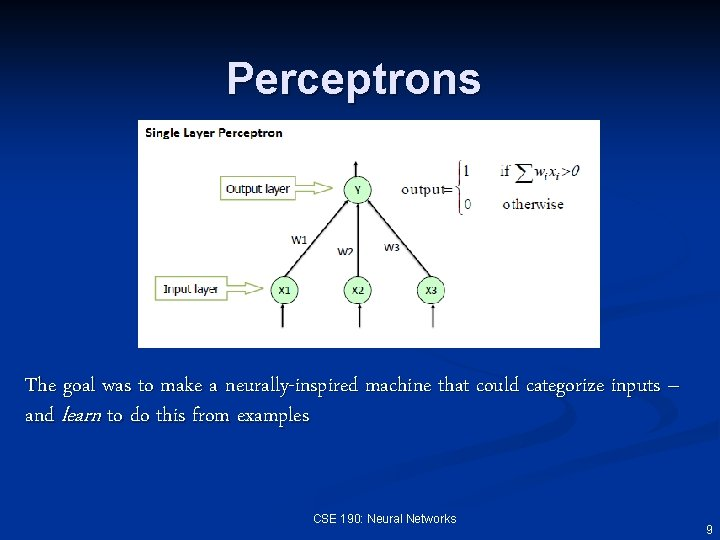Perceptrons The goal was to make a neurally-inspired machine that could categorize inputs – and learn to do this from examples CSE 190: Neural Networks 9Learning: A bit of history n Rosenblatt (1962) discovered a learning rule for perceptrons called the perceptron convergence procedure. n Guaranteed to learn anything computable (by a two-layer perceptron) n Unfortunately, not everything was computable (Minsky & Papert, 1969) 9/25/15 CSE 190: Neural Networks 10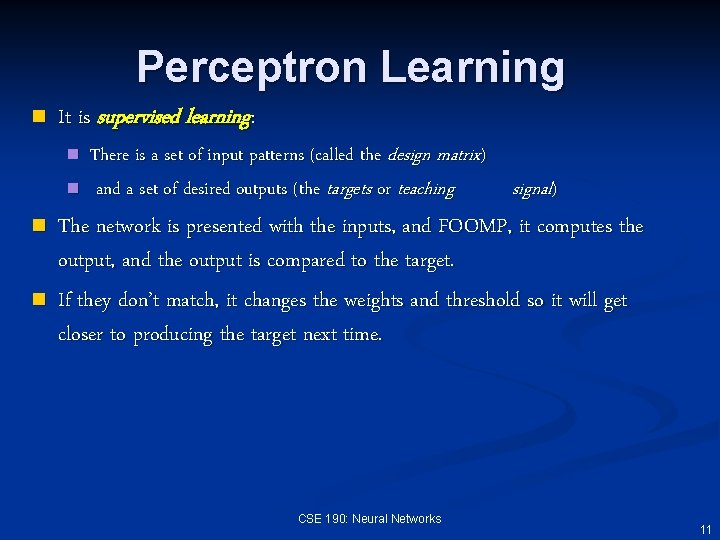Perceptron Learning n It is supervised learning: n n There is a set of input patterns (called the design matrix) and a set of desired outputs (the targets or teaching signal) The network is presented with the inputs, and FOOMP, it computes the output, and the output is compared to the target. If they don’t match, it changes the weights and threshold so it will get closer to producing the target next time. CSE 190: Neural Networks 11Perceptron Learning First, get a training set - let’s choose OR Four “patterns”: INPUT TARGET 00 0 01 1 10 1 11 1 This is the design matrix (you don’t need to know that, but it makes you sound smarter at conferences… CSE 190: Neural Networks 12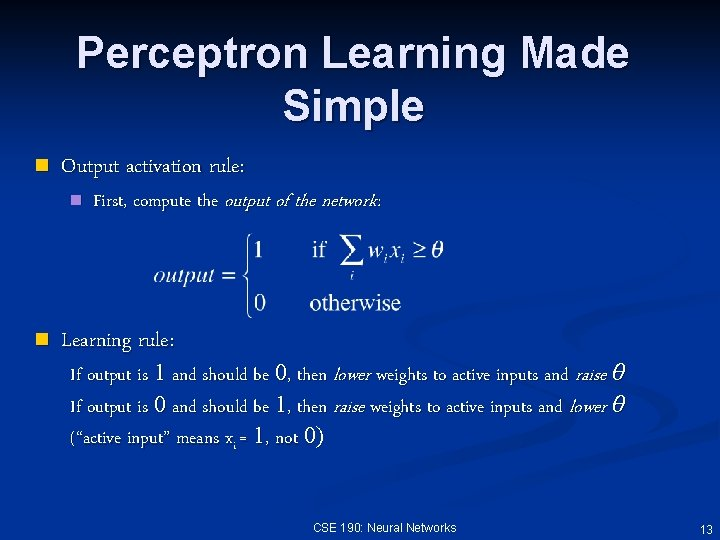Perceptron Learning Made Simple n Output activation rule: n n First, compute the output of the network: Learning rule: If output is 1 and should be 0, then lower weights to active inputs and raise θ If output is 0 and should be 1, then raise weights to active inputs and lower θ (“active input” means xi = 1, not 0) CSE 190: Neural Networks 13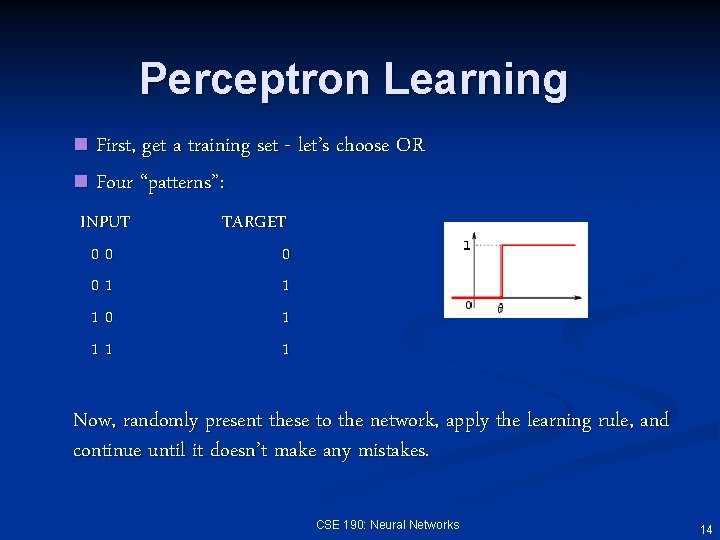Perceptron Learning First, get a training set - let’s choose OR n Four “patterns”: n INPUT 00 01 10 11 TARGET 0 1 1 1 Now, randomly present these to the network, apply the learning rule, and continue until it doesn’t make any mistakes. CSE 190: Neural Networks 14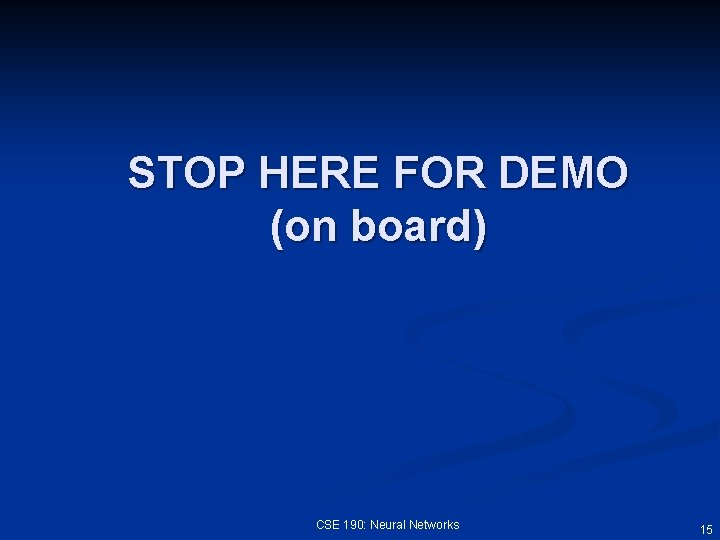STOP HERE FOR DEMO (on board) CSE 190: Neural Networks 15Characteristics of perceptron learning n Supervised learning: Gave it a set of input-output examples for it to model the function (a teaching signal) n Error correction learning: only corrected it when it is wrong (never praised! ; -)) n Random presentation of patterns. n Slow! Learning on some patterns ruins learning on others. 9/25/15 CSE 190: Neural Networks 16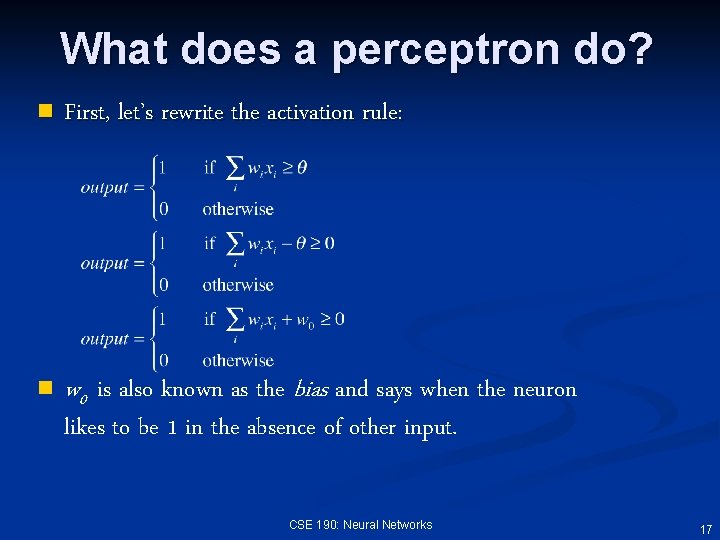What does a perceptron do? n First, let’s rewrite the activation rule: n w 0 is also known as the bias and says when the neuron likes to be 1 in the absence of other input. CSE 190: Neural Networks 17What does a perceptron do? n One more step: n Here we simply are rewriting the expression as a function: n Notation: BOLD w and BOLD x are vectors. CSE 190: Neural Networks 18What does a perceptron do? n n Ok, so, now our activation rule is: Now, we call y(x)=0 the decision boundary – where y(x) changes This now has a simple geometrical interpretation: y(x)=0 is a d-1 dimensional hyperplane in a d-dimensional input space So for 2 D, it is a line: CSE 190: Neural Networks 19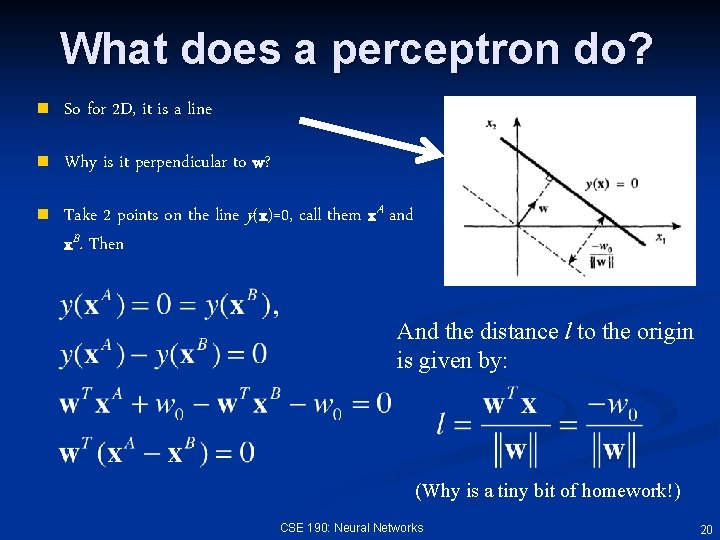What does a perceptron do? n So for 2 D, it is a line n Why is it perpendicular to w? n Take 2 points on the line y(x)=0, call them x. A and x. B. Then And the distance l to the origin is given by: (Why is a tiny bit of homework!) CSE 190: Neural Networks 20